# Blend effect

Use the blend effect to combine 2 images. This effect has 26 blend modes.

The CLSID for this effect is CLSID_D2D1Blend.

## Blending examples

Here is an example image of every blend mode of the blend effect. A full list of the blend modes and the corresponding mode properties are in the next sectionHere is another example using the exclusion mode.

Before image 1Before image 2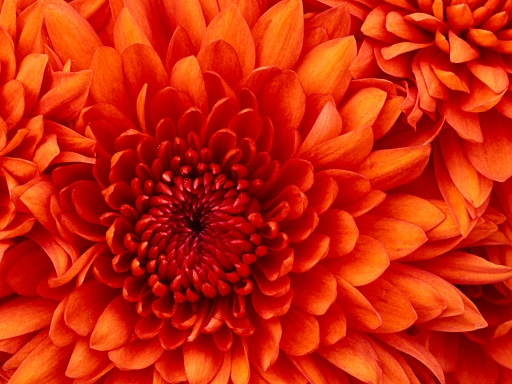After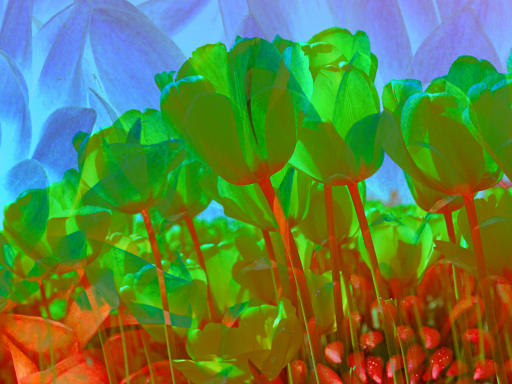``````ComPtr<ID2D1Effect> blendEffect;
m_d2dContext->CreateEffect(CLSID_D2D1Blend, &blendEffect);

blendEffect->SetInput(0, bitmap);
blendEffect->SetInput(1, bitmapTwo);
blendEffect->SetValue(D2D1_BLEND_PROP_MODE, D2D1_BLEND_MODE_EXCLUSION);

m_d2dContext->BeginDraw();
m_d2dContext->DrawImage(blendEffect.Get());
m_d2dContext->EndDraw();
``````

## Effect properties

Display name and index enumeration Description
Mode
D2D1_BLEND_PROP_MODE
The blend mode used for the effect. See Blend modes for more info. The type is D2D1_BLEND_MODE.
The default value is D2D1_BLEND_MODE_MULTIPLY.

## Blend modes

The table here shows all the blend modes of this effect. The helper functions necessary to compute the output of the effect are in the next section.

Color: OPRGB = f(FRGB, BRGB) * FA * BA + FRGB * FA * (1 - BA) + BRGB * BA * (1 - FA)

Alpha: OA = FA * (1 - BA) + BA

Where:

• OPRGB is the pre-multiplied output color
• OA is Output Alpha
• BRGB is the un-pre-multiplied destination color
• BA is destination alpha
• FRGB is the un-pre-multiplied source color
• FA is source alpha
• f(SRGB, DRGB) is a blend function that varies per-blend-mode

Some of the blend modes require conversion to and from the hue, saturation, luminosity (HSL) color space to RGB.

Enumeration Equation
D2D1_BLEND_MODE_DARKEN Basic blend formula for alpha only.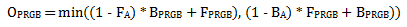D2D1_BLEND_MODE_MULTIPLY Basic blend formula for alpha only.D2D1_BLEND_MODE_COLOR_BURN Basic blend formulas with f(FRGB, BRGB) =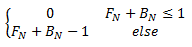D2D1_BLEND_MODE_LINEAR_BURN Basic blend formulas with f(FRGB, BRGB) =D2D1_BLEND_MODE_DARKER_COLOR Basic blend formula for alpha only.D2D1_BLEND_MODE_LIGHTEN Basic blend formula for alpha only.D2D1_BLEND_MODE_SCREEN Basic blend formula for alpha only.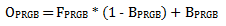D2D1_BLEND_MODE_COLOR_DODGE Basic blend formulas with f(FRGB, BRGB) =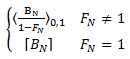D2D1_BLEND_MODE_LINEAR_DODGE Basic blend formulas with f(FRGB, BRGB) =D2D1_BLEND_MODE_LIGHTER_COLOR Basic blend formula for alpha only.D2D1_BLEND_MODE_OVERLAY Basic blend formulas with f(FRGB, BRGB) =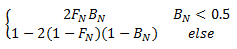D2D1_BLEND_MODE_SOFT_LIGHT Basic blend formulas with f(FRGB, BRGB) =D2D1_BLEND_MODE_HARD_LIGHT Basic blend formulas with f(FRGB, BRGB) =D2D1_BLEND_MODE_VIVID_LIGHT Basic blend formulas with f(FRGB, BRGB) =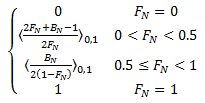D2D1_BLEND_MODE_LINEAR_LIGHT Basic blend formulas with f(FRGB, BRGB) =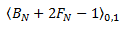D2D1_BLEND_MODE_PIN_LIGHT Basic blend formulas with f(FRGB, BRGB) =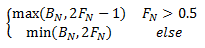D2D1_BLEND_MODE_HARD_MIX Basic blend formulas with f(FRGB, BRGB) =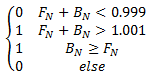D2D1_BLEND_MODE_DIFFERENCE Basic blend formulas with f(FRGB, BRGB) = abs(FRGB - BRGB)
D2D1_BLEND_MODE_EXCLUSION Basic blend formulas with f(FRGB, BRGB) = FRGB + BRGB 2 * FRGB * BRGB
D2D1_BLEND_MODE_HUE Basic blend formula for alpha only.D2D1_BLEND_MODE_SATURATION Basic blend formula for alpha only.D2D1_BLEND_MODE_COLOR Basic blend formula for alpha only.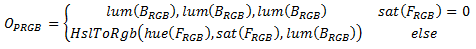D2D1_BLEND_MODE_LUMINOSITY Basic blend formula for alpha only.D2D1_BLEND_MODE_DISSOLVE Given:
• A scene coordinate XY for the current pixel
• A deterministic pseudo-random number generator rand(XY) based on seed coordinate XY, with unbiased distribution of values from [0, 1]D2D1_BLEND_MODE_SUBTRACT Basic blend formula for alpha only.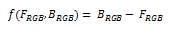D2D1_BLEND_MODE_DIVISION Basic blend formula for alpha only.Note

For all Blend modes, the output value is premultiplied and clamped to the range [0, 1].

## HSL color space conversions

The luminosity component is computed using the RGB weights here:

• kR = 0.30
• kG = 0.59
• kB = 0.11

### Converting from RGB to HSLThis places S and L in the range [0.0, 1.0] and H in the range [-1.0, 5.0].

### Converting from HSL to RGB

To convert the other way we use the inverse of the previous calculations.

If S = 0 then R = G = B = L

Otherwise there are six hue-dependant cases:

If H is greater than 0, the values are in the red/magenta sector where R > B > G.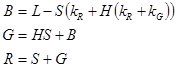If H is greater than or equal to 0 and less than 1, the values are in the red/yellow sector where R > G > B.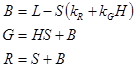If H is greater than or equal to 1 and less than 2, the values are in the yellow/green sector where G > R > B.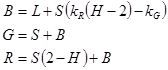If H is greater than or equal to 2 and less than 3, the values are in the green/cyan sector where G > B > R.If H is greater than or equal to 3 and less than 4, the values are in the cyan/blue sector where B > G > R.If H is greater than or equal to 4, the values are in the blue/magenta sector where B > R > G.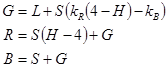Because the blending modes make arbitrary combinations of HSL components from two different colors, it is common for the converted RGB value to be out-of-gamut, that is, one or more channel components may be outside the legal range of [0.0, 1.0]. These colors are brought back into gamut by minimally reducing the saturation, while preserving both hue and luminosity:## Output bitmap

The output bitmap for this effect is always the size of the union of the two input images.

## Sample code

For an example of this effect, download the Direct2D composite effect modes sample.

## Requirements

Requirement Value
Minimum supported client Windows 8 and Platform Update for Windows 7 [desktop apps | Windows Store apps]
Minimum supported server Windows 8 and Platform Update for Windows 7 [desktop apps | Windows Store apps]